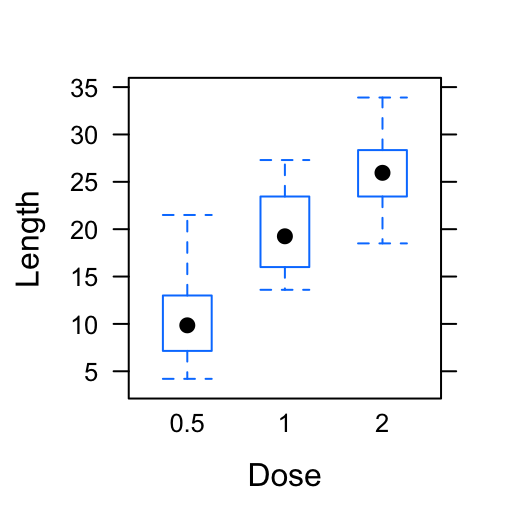# Lattice Graphs

Previously, we described the essentials of R programming and provided quick start guides for importing data into R. We also showed how to visualize data using R base graphs.

Here, we’ll present the basics lattice package, which is a powerful and elegant data visualization system that aims to improve on base R graphs.

1. Launch RStudio as described here: Running RStudio and setting up your working directory

2. Prepare your data as described here: Best practices for preparing your data and save it in an external .txt tab or .csv files

3. Import your data into R as described here: Fast reading of data from txt|csv files into R: readr package.

Briefly, if your data is saved in an external .txt tab or .csv files, use the following script to import the data into R:

``````# If .txt tab file use this:
# or if .csv file:

In the following sections, we’ll use R built-in data sets.

``````# Install
install.packages("lattice")
library("lattice")``````

# Main functions in the lattice package

Function Description
xyplot() Scatter plot
splom() Scatter plot matrix
cloud() 3D scatter plot
stripplot() strip plots (1-D scatter plots)
bwplot() Box plot
dotplot() Dot plot
barchart() bar chart
histogram() Histogram
densityplot Kernel density plot
qqmath() Theoretical quantile plot
qq() Two-sample quantile plot
contourplot() 3D contour plot of surfaces
levelplot() False color level plot of surfaces
parallel() Parallel coordinates plot
wireframe() 3D wireframe graph

Note that, other functions (ecdfplot() and mapplot()) are available in the latticeExtra package.

# xyplot(): Scatter plot

• R function: The R function xyplot() is used to produce bivariate scatter plots or time-series plots. The simplified format is as follow:
``xyplot(y ~ x, data)``
• Data set: mtcars
``````my_data <- iris
``````##   Sepal.Length Sepal.Width Petal.Length Petal.Width Species
## 1          5.1         3.5          1.4         0.2  setosa
## 2          4.9         3.0          1.4         0.2  setosa
## 3          4.7         3.2          1.3         0.2  setosa
## 4          4.6         3.1          1.5         0.2  setosa
## 5          5.0         3.6          1.4         0.2  setosa
## 6          5.4         3.9          1.7         0.4  setosa``````
• Basic scatter plot: y ~ x
``````# Default plot
xyplot(Sepal.Length ~ Petal.Length, data = my_data)````````````# Color by groups
xyplot(Sepal.Length ~ Petal.Length, group = Species,
data = my_data, auto.key = TRUE)````````````# Show points ("p"), grids ("g") and smoothing line
# Change xlab and ylab
xyplot(Sepal.Length ~ Petal.Length, data = my_data,
type = c("p", "g", "smooth"),
xlab = "Miles/(US) gallon", ylab = "Weight (1000 lbs)")``````• Multiple panels by groups: y ~ x | group
``````xyplot(Sepal.Length ~ Petal.Length | Species,
group = Species, data = my_data,
type = c("p", "smooth"),
scales = "free")``````# cloud(): 3D scatter plot

• Data set: iris
``````my_data <- iris
``````##   Sepal.Length Sepal.Width Petal.Length Petal.Width Species
## 1          5.1         3.5          1.4         0.2  setosa
## 2          4.9         3.0          1.4         0.2  setosa
## 3          4.7         3.2          1.3         0.2  setosa
## 4          4.6         3.1          1.5         0.2  setosa
## 5          5.0         3.6          1.4         0.2  setosa
## 6          5.4         3.9          1.7         0.4  setosa``````
• Scatter 3D plot: z ~ x * y
``````# Basic 3D scatter plot
cloud(Sepal.Length ~ Sepal.Length * Petal.Width,
data = iris)````````````# Color by groups; auto.key = TRUE to show legend
cloud(Sepal.Length ~ Sepal.Length * Petal.Width,
group = Species, data = iris,
auto.key = TRUE)``````# Box plot, Dot plot, Strip plot

• Data set: ToothGrowth
``````ToothGrowth\$dose <- as.factor(ToothGrowth\$dose)
``````##    len supp dose
## 1  4.2   VC  0.5
## 2 11.5   VC  0.5
## 3  7.3   VC  0.5
## 4  5.8   VC  0.5
## 5  6.4   VC  0.5
## 6 10.0   VC  0.5``````
• Basic plot: Plot len by dose
``````# Basic box plot
bwplot(len ~ dose,  data = ToothGrowth,
xlab = "Dose", ylab = "Length")
# Violin plot using panel = panel.violin
bwplot(len ~ dose,  data = ToothGrowth,
panel = panel.violin,
xlab = "Dose", ylab = "Length")

# Basic dot plot
dotplot(len ~ dose,  data = ToothGrowth,
xlab = "Dose", ylab = "Length")
# Basic stip plot
stripplot(len ~ dose,  data = ToothGrowth,
jitter.data = TRUE, pch = 19,
xlab = "Dose", ylab = "Length")``````• Plot with multiple groups: Additional argument layout is used: c(3, 1) specifying the number of column and row, respectively
``````# Box plot
bwplot(len ~ supp | dose,  data = ToothGrowth,
layout = c(3, 1),
xlab = "Dose", ylab = "Length")
# Violin plot
bwplot(len ~ supp | dose,  data = ToothGrowth,
layout = c(3, 1), panel = panel.violin,
xlab = "Dose", ylab = "Length")
# Dot plot
dotplot(len ~ supp | dose,  data = ToothGrowth,
layout = c(3, 1),
xlab = "Dose", ylab = "Length")
# Strip plot
stripplot(len ~ supp | dose,  data = ToothGrowth,
layout = c(3, 1), jitter.data = TRUE,
xlab = "Dose", ylab = "Length")``````# Density plot and Histogram

• Basic plots
``````densityplot(~ len, data = ToothGrowth,
plot.points = FALSE)
histogram(~ len, data = ToothGrowth,
breaks = 20)``````• Plot with multiple groups
``````densityplot(~ len, groups = dose, data = ToothGrowth,
plot.points = FALSE, auto.key = TRUE)``````# Infos

This analysis has been performed using R statistical software (ver. 3.2.4).